Courses

Test: Ratio And Proportion, Indices, Logarithms - 3

40 Questions MCQ Test Quantitative Aptitude for CA CPT | Test: Ratio And Proportion, Indices, Logarithms - 3

Description
Attempt Test: Ratio And Proportion, Indices, Logarithms - 3 | 40 questions in 40 minutes | Mock test for CA Foundation preparation | Free important questions MCQ to study Quantitative Aptitude for CA CPT for CA Foundation Exam | Download free PDF with solutions
QUESTION: 1

Solution:
QUESTION: 2

Find the value of [log10 25 - log10(23) + log10(4)2]

Solution:

= [log10 25 - log10(23) + log10(4)2]
= (1.397940009 - 0.903089987 +  1.204119983)

= 1.698970005

QUESTION: 3

If 2x-2x-1=4 then xx is equal to :

Solution:

2x-2x-1 =4 , then taking common 2x-1
2x-1(2-1) = 4 , 2x-1 =4 ,
2x-1=22, simplify
x-1 = 2, x = 3 then
x= 3= 27
hence 27 is the required answer

QUESTION: 4

What must be added to each term of the ratio 49:68, so that it becomes 3:4?

Solution:
QUESTION: 5

If A, B and C started a business by investing Rs. 1,26,000, Rs. 84,000 and Rs. 2,10,000. If at the end of the year profit is Rs. 2,42,000 then the share of each is:

Solution:

QUESTION: 6

If A:B = 2:3, B:C = 4:5 and C:D = 6:7, then find the value of A:B:C:D

Solution: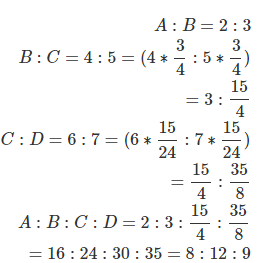QUESTION: 7

Log4 (x2+x) - log4 (x+1) = 2. Find x

Solution: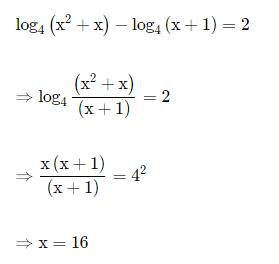QUESTION: 8

Fourth proportional to x, 2x, (x+1) is:

Solution:
QUESTION: 9

If x=31/3+3-1/3 then find value of 3x3-9x

Solution:
QUESTION: 10

If loga b + loga c=0 then

Solution:
QUESTION: 11

The value of log2 (log5 625) is :​

Solution:

Let log5 625 = x.

Then, 5x = 625 = 54 or x = 4.

Let log2(log5 625) = y.

Then, log2 4 = y or 2y= 4 = 22 or y = 2.

∴ log2(log5 625) = 2.

QUESTION: 12

If A:B=2:5, then (10A+3B):(5A+2B) is equal to

Solution:

A/B = 2/5

so in (10A+3B)/(5A+2B)

divide both numerator and denominator by B

(10A/B +3)/(5A/B +2)

put A/B value

this becomes (10 * 2/5 + 3)/(5 * 2/5 + 2)

=7/4 = 7:4

QUESTION: 13

Find the sub-duplicate ratio of 81 : 64 ?

Solution:

Required sub - duplicate ratio of 81 : 64 = √81 : √64 = 9 : 8

QUESTION: 14

The sub duplicate ratio of 25:36 is

Solution:
QUESTION: 15

The ratio compounded of 2:3, 9:4, 5:6 and 8:10 is

Solution:
QUESTION: 16

The sub triplicate ratio of 8:27 is

Solution:
QUESTION: 17

The triplicate ratio of 2:3 is

Solution:
QUESTION: 18

The student of two classes are in the ratio 5:7, if 10 students left from each class, the remaining students are in the ratio of 4:6 then the number of students in each class is:

Solution:
QUESTION: 19

The ratio of two quantities is 3:4. If the antecedent is 15, the consequent is

Solution:
QUESTION: 20

The ratio compounded of 4:9, the duplicate ratio of 3:4, the triplicate ratio of 2:3 and 9:7is

Solution:

Step-by-step explanation:

The duplicate ratio of 3:4 is 3^2/4^2 = 9/16.

The triplicate ratio of 2:3 is 2^3/3^3 = 8/27.

Now we have 4 ratios: 4:9, 9:16, 8:27 and 9:7.

We have to calculate the ratio compounded of all these ratios.

That will be (4 x 9 x 8 x 9)/(9 x 16 x 27 x 7) = 2/21.

QUESTION: 21

The duplicate ratio of 3:4 is

Solution:
QUESTION: 22

The ratio compounded of 4:9 and the duplicate ratio of 3:4 is

Solution:

Option ( a) 1:4  is right.

Explanation:-
Duplicate ratio of 3:4 , will be the square of the ratio, so this will be  9:16

And the compounded ratio will be product of two ratios:-

4:9 * 9:16 => 1:4

( 9 will be cancelled and 16 by 4 )

QUESTION: 23

The recurring decimal 2.7777………….can be expressed as

Solution:
QUESTION: 24

The ratio of the quantities is 5:7. If the consequent of its inverse ratio is 5, the antecedent is

Solution:
QUESTION: 25

The angles of a triangle are in ratio 2:7:11. The angles are

Solution:
QUESTION: 26

If p:q =2:3 and x:y = 4:5, then the value of 5px + 3qy: 10px + 4qy is

Solution:

Given as :

The ratio are as follows

p : q = 2 : 3

Let The value of p = 2 a ,

Let The value of q = 3 a

And

x : y = 4 : 5

Let The value of x = 4 b

Let The value of y = 5 b

Now, According to question

Let 5 p x + 3 q y : 10 p x + 4 q y  = m : n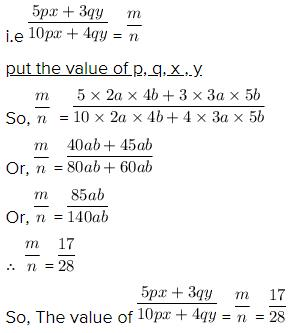QUESTION: 27

Two numbers are in the ratio 2:3. If 4 be subtracted from each, they are in the ratio 3:5. The numbers are

Solution:

Two numbers are in the ratio 2:3.

Let the two numbers are 2x and 3x.

If 4 be subtracted from each, they are in the ratio 3:5.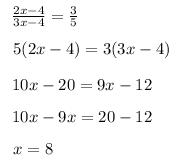The value of x is 8.

The two numbers are

2x = 2 * 8 = 16

3x = 3 * 8 = 24

Therefore the two numbers are 16 and 24.

QUESTION: 28

If x:y = 3:4, the value of (x2y+xy2):(x3 +y3) is

Solution:

(x2y+xy2):(x3 +y3) divide boht numerator and denominator by y3

(x2/y2 + x/y) / ((x/y)3 + 1)

put value of x/y = 3/4

(9/16 + 3/4) / (27/64 + 1)

= 12/13

QUESTION: 29

P, Q and R are three cities. The ratio of average temperature between P and Q is 11:12 and that between P and R is 9:8. The ratio between the average temperature of Q and R is

Solution:
QUESTION: 30

The ratio of two numbers is 7:10 and their difference is 105. The numbers are

Solution:

We don't know the numbers so let it be xX

7x,10x and they said that the difference of two numbers is 105

10x-7x=105

3x=105

X=105/3

X=35

So the numbers are 245,350

QUESTION: 31

Daily earnings of two persons are in the ratio 4:5 and their daily expenses are in the ratio 7:9. If each saves Rs. 50 per day, their daily incomes in Rs. are

Solution:
QUESTION: 32

The number which when subtracted from each of the terms of the ratio 19:31 reducing it to 1:4 is

Solution:
QUESTION: 33

If 2s:3t is the duplicate ratio of 2s-p:3t-p then

Solution:
QUESTION: 34

The ratio compounded of duplicate ratio of 4:5, triplicate ratio of 1:3, sub duplicate ratio of 81: 256 and sub triplicate ratio of 125: 512 is

Solution:

dupicate ratio of 4:5 = 16:25

triplicate ratio of 1:3 = 9:27

sub-duplicate ratio of 81:256 = 9:16

sub-triplicate ratio of 125:512 = 5:8

﻿﻿compounded ratio=720:86400 = 1:120

QUESTION: 35

The ratio between the speeds of two trains is 7: 8. If the second train runs 400 kms in 4 hours, then the speed of the first train is ?

Solution:

Let the speed of two trains be 7x and 8x km/hr.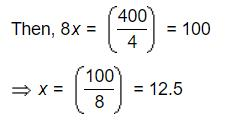Speed of first train = (7 x 12.5) km/hr = 87.5 km/hr.

QUESTION: 36

Division of Rs. 324 between X and Y is in the ratio 11:7. X & Y would get Rupees

Solution:
QUESTION: 37

If a : b = 3:4, the value of (2a+3b) : (3a+4b) is

Solution:

a/b=3/4
So,

[(2 x 3k)+(3 x 4k)]/[(3 x 3k)+(4 x 4k)]

= (6k+12k)/(9k+16k)

=18/25.

QUESTION: 38

Anand earns Rs. 80 in 7 hours and Promod Rs. 90 in 12 hours. The ratio of their earnings is

Solution:

Earning of Anand in 1 hour=80/7
earning of promod in 1 hour 90/12
so ratio of their earnings =(80/7)/90/12=80*12/90*7=96/63=32/21

QUESTION: 39

If x/2=y/3=z/7, then the value of (2x-5y+4z)/2y is

Solution:
QUESTION: 40

The ratio of market prices of wheat and paddy is 2:3 and the ratio of quantities consumed in a family is 5:4. Find the ratio of expenditure of wheat and paddy.

Solution: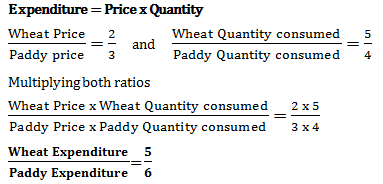Use Code STAYHOME200 and get INR 200 additional OFF Use Coupon Code

Track your progress, build streaks, highlight & save important lessons and more!

Similar ContentRelated tests